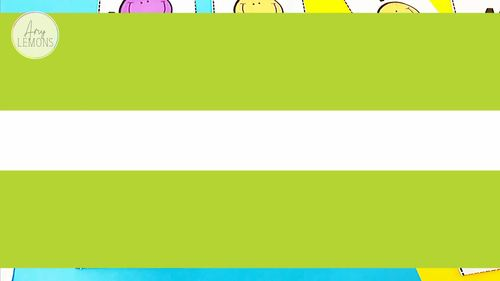# Addition and Subtraction Math Centers

Rated 4.9 out of 5, based on 1977 reviews
2k Ratings;
1st - 2nd
Subjects
Resource Type
Formats Included
• PDF
Pages
46 pages
Report this resource to TPT

#### What educators are saying

I love your resources! You have the perfect balance between creative, cute and intentional. This keeps my students engaged while reinforcing their skills. Thanks! :)
This resource helped my students practice Math skills in an engaging way and were a great way to add some fun into our lessons! I absolutely love all of the activities!!!
##### Also included in
1. This Bundle contains the following three units! If you already own these units, please do not purchase again! Learning Our Addition StrategiesLearning Our Subtraction StrategiesAddition and Subtraction Math Stations Math Station Description: 3-8: Add and Match- Students match the addition senten
Price \$15.60Original Price \$19.50Save \$3.90

### Description

Wanting your students to practice their fact fluency without just using flashcards? These addition and subtraction math stations will engage your students to where they won't even realize they are learning! There are 5 addition and 5 subtraction stations included. I made these stations so they would be easy to prep! All stations are color (but not ink-heavy) and can be printed in grayscale! Here's all the details:

Add and Match- Students match the addition sentence to the sum. (sums up to 18)

Subtract and Match- Students match the subtraction sentence to the difference. (subtracting from 12 and below)

Spin and Add- Students spin both spinners to find the addends. Then, students add the two numbers together to find the sum. (sums up to 17)

Spin and Subtract- Students spin both spinners. Then, students subtract the number from Spinner B from the number from Spinner A. Students write the difference. (subtracting from 12 and below)

Find the Missing Addend- Students match the missing addend with the addition sentence. (sums up to 16)

Find the Missing Number- Students match the missing number with the subtraction sentence. (subtracting from 15 and below)

Addition Puzzles- Students match the 2 addends to the sum to complete the puzzles. (sums up to 17)

Minus 1, 2, 3- Students subtract 1, 2, and 3 from each number card. (subtracting from 17 and below)

Addition BINGO- with sums up to 24

Subtraction BINGO- with differences up to 23

20 or Bust

Pair these with my Addition Strategies and Subtraction Strategies for extra fun!

Looking for more math stations? Check out the units below:

Number Knowledge

Easy as 1-2-3 Centers for Back to School Math Stations

Fall Math Stations

Spring Math Stations

Warm Me Up! Winter Math Stations

Hi, Valentine! ELA and Math Stations

Spin on! 10 Math Stations with Spinners

3 Digit Addition and Subtraction Centers

Some Bunny Loves You Easter Math Stations

It's a Penguin Party Penguin Math Stations

Math Stations Bundle

Total Pages
46 pages